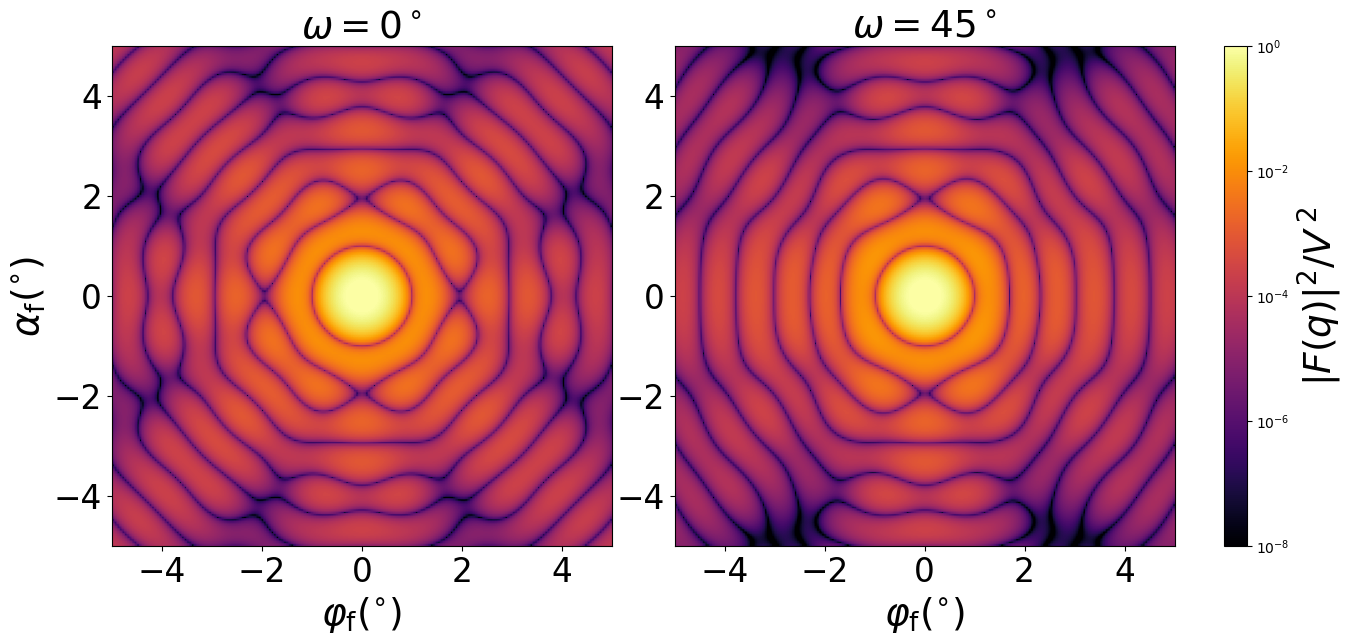### CantellatedCube

A cube with truncated edges and vertices as in Fig 7 of [Croset 2017]. Can also be obtained by cantellating an octahedron. Also known as Rhombicuboctahedron.#### Constructor

CantellatedCube(L, t)


Parameters:

• L, length of the full cube
• t, side length of the birectangular prisms removed from the cube’s edges

Constraint:

$t \le L/2$

#### Usage

As for any other Form factor.

#### Implementation

Class CantellatedCube inherits from the interface class IFormFactor .

Form factor computation is based on the generic form factor of a polyhedron with inversion symmetry provided by libformfactor .

Volume has been validated against $$V= L^3 - 6Lt^2 + \dfrac{16}{3} t^3.$$

Scattering by uncorrelated, oriented cantellated cubes for horizontal incidence. Rotation around $z$ axis:Generated by Examples/ff/CantellatedCube.py .

#### History

Introduced in BornAgain 1.17 (Python only). Motivated by [Croset 2017].